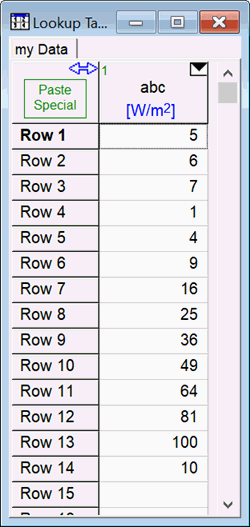Contents - Index

\$CopyToLookup

The \$CopyToLookup directive (Professional version) provides a simple way of moving selected variables in the main program or in Functions, Procedures, or Subprograms to a specified column in a specified Lookup table.  This directive provides a simpler and faster alternative to using the Lookup command on the left side of an equation in a Function or Procedure as explained in the use of the Lookup function.  However, the \$CopyToLookup directive is only implemented in the Professional version.

The format of the \$CopyToLookup Directive is

\$CopyToLookup  /T  /C  /R    'TableName',  'ColumnName', StartRow,  Var1, Var2, Var3[1..N]

'Tablename' is the name of the Lookup table that the data will be written to.  'Tablename' can be a string constant surrounded by single quotes as shown or a string variable that has been previously defined.

'ColumnName' is the name of the column in the Lookup table that the data will be written to.  'ColumnName' can be a string constant surrounded by single quotes as shown or a string variable that has been previously defined.  In addition, the units for the column can be specified by following the column name with a backslash (\) and then then the units.

StartRow is the row for which the first data item will be written.  Each data item is written in a successive row.  StartRow can be an integer value (e.g., 1)  or a previously defined EES variable.

Var1, Var2, Var3[1..N] are EES variables that appear in the main program, Function, Procedure, or Subprogram that the \$CopyToLookup directive is located within.  Each variable will appear in a successive row of the specified column.  Note that array range notation can be used to specify that a range of array variables.  The limits can be integers or previously defined EES variables, e.g., N.

/T is optional.  If present, it will create a new Lookup table named 'TableName' if there is no existing Lookup table with this name. (Without this option, the Lookup table must already exist.)

/C is optional.  If present, it will add a column named 'ColumnName' to the Lookup table named 'TableName' if a column with this name does not already exist.

/R is optional.  If present, this option will add rows to the Lookup table as needed to ensure that all of the data specified data are included in the Lookup table.

The \$CopyToLookup directive can be placed anywhere in the Equations Window, including within Functions, Procedures, and Subprograms.  Multiple \$CopyToLookup directives are permitted.

Example:

"Start EES and enter the following equations:  Do not create a Lookup table as one will be created automatically with 50 rows."

x=5

y=6

z=7

N=10

duplicate i=1,N

x[i]=i^2

end

N\$='abc\W/m^2'                  "column name is set to abc with units W/m^2"

X\$='my Data'

\$copytoLookup /T /R /C X\$  N\$   1  X,y,z,x[1..N],N

After solving, a lookup table named 'my Data' having one column named 'abc' will be generated and it will contain the data shown in the figure.See also:    Lookup